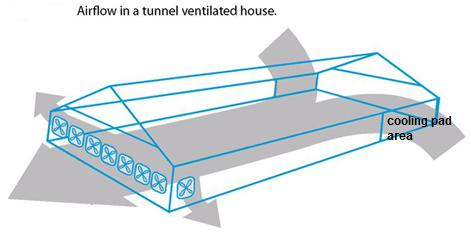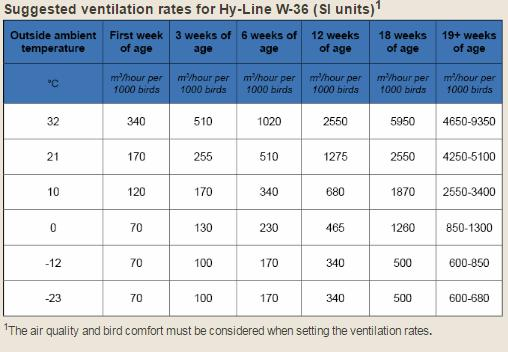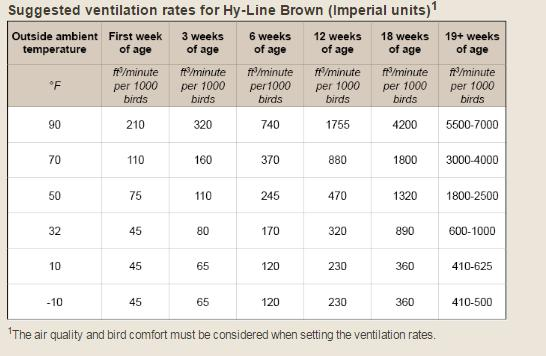# Calculation of Poultry House Ventilation## Calculation of Poultry House Ventilation

### Air exchange area and calculation of fans in a poultry house

Air exchange area  m3/minute = Length width height

For example

Air exchange area m3/minute =  120m×12m×03m

 Air exchange area m3/minute =  4320 m3

## No. of fan needed for air exchange  =

Air volume m3/minute   Air exchange capacity of Fan

Standards

1. Fan with diameter of 1.2m = 566 m3/minute
2. Fan with diameter of 1.0m = 283 m3/minute
3. Fan with diameter of 91 cm= 255 m3/minute

For example

No. of fan needed for air exchange = Air volume m3/minute ÷ Air exchange capacity of Fan

No. of fan needed for air exchange =  4320 ÷ 566 =7.6 or 8 Fans

 No. of fan needed for air exchange = 08 fans

## Calculation of space  for Cooling Pad  in poultry house(m2) =

(Fan air exchange Capacity m3/minute × Number of fans)÷ Air Speed through cooling pad m3/minute

Standards

1. For 100mm (10cm or 4-in) thick Pad,
use     ( 1.27m/s  or 76m/minute )(250fpm)

2. For 150mm (15cm or 6-in) thick Pad,
use   ( 1.91m/s or  114/minute ) (375fpm)

For example

= (Fan air exchange Capacity m3/minute × No. of fans)÷ (Air Speed through cooling pad m3/minute)

Cooling  Pad area = (566  × 8 )÷114 = 39.7m2  Or  40m

*It means  20m2 (2m in width and 10 m in length) on each side of the house

 Cooling  Pad area in m2 = 40m2

## The number of fans Needed to exchange required air velocity

=(Required Air velocity m/s  ) ÷{  Fan capacity ÷ (width × Height ×60) }

Standard  Air velocity

Rearing      ˃ 2.03 meter per second (m/s) OR 400 Feet per minute (fpm)

Production ˃ 2.54 meter per second (m/s) OR 500 Feet per minute (fpm)

For example

The number of fans Needed to exchange required air velocity

(Required Air velocity m/s  ) ÷{  Fan capacity ÷ (width × Height ×60) }

No. of fans Needed to exchange required air velocity  =(2.03) ÷ { 566 ÷(12×03×60} =7.8 fans

 No. of fans Needed to exchange required air velocity  = Fans 8

## Minimum Ventilation Calculation For Fan Timer Setting

Standards

Minimum ventilation per bird

 Age Cubic meter per hour(CMH/Bird) Cubic feet per minute(CFM/Bird) 01-08 weeks 0.16 0.10 09-15 weeks 0.42 0.25 16-35 weeks 0.59 0.35 35- depletion 0.76 0.45

For example

Bird Age = 20 weeks

Number of Birds = 10,000

Minimum ventilation fan = 1 × 91 cm =255 m3/minute × 60 =( 15300m3/hr )

Using a 5 minutes cycle timer

Calculate the minimum Ventilation ?

Step:1

Ventilation rate = (Minimum ventilation per bird) ×( Number of Birds)

Ventilation rate= (0.59 cmh) ×(10,000) = 5,900 cmh (cubic meter per houre)

## Percentage time the fans needed to be run for

Step:2

Percentage time = (Total ventilation needed) ÷ (Total capacity of fans used)

Percentage time =(5,900cmh) ÷ (15,300) =0.39 or 39%

 Percentage time =39%

*It means if a 5 minute timer is used, the run timer required would be then 39% of  5 minutes ,or 117 seconds

( or fan should be 24 sec on and 36 sec off for each minute)

For example

Bird Age = 01 weeks

Number of Birds = 10,000

Minimum ventilation fan = 1 × 91 cm =255 m3/minute × 60 =( 15300m3/hr )

Using a 5 minutes cycle timer

Calculate the minimum Ventilation ?

Step:1

Ventilation rate = (Minimum ventilation per bird) ×( Number of Birds)

Ventilation rate= (0.16 cmh) ×(10,000) = 1600 cmh (cubic meter per houre)

## Percentage time the fans needed to be run for

Step:2

Percentage time = (Total ventilation needed) ÷ (Total capacity of fans used)

Percentage time =(1600cmh) ÷ (15,300) =0.10 or 10%

 Percentage time =10%

*Assuming a 05 minute cycle timer, the run Timer required would then be 10 % of 05 minutes ,or30`seconds

## Ventilation standers for Layers###### By Dr.Syed Irfan Hussain
(Visited 555 times, 1 visits today)
Share

This site uses Akismet to reduce spam. Learn how your comment data is processed.